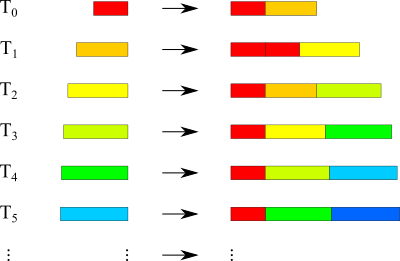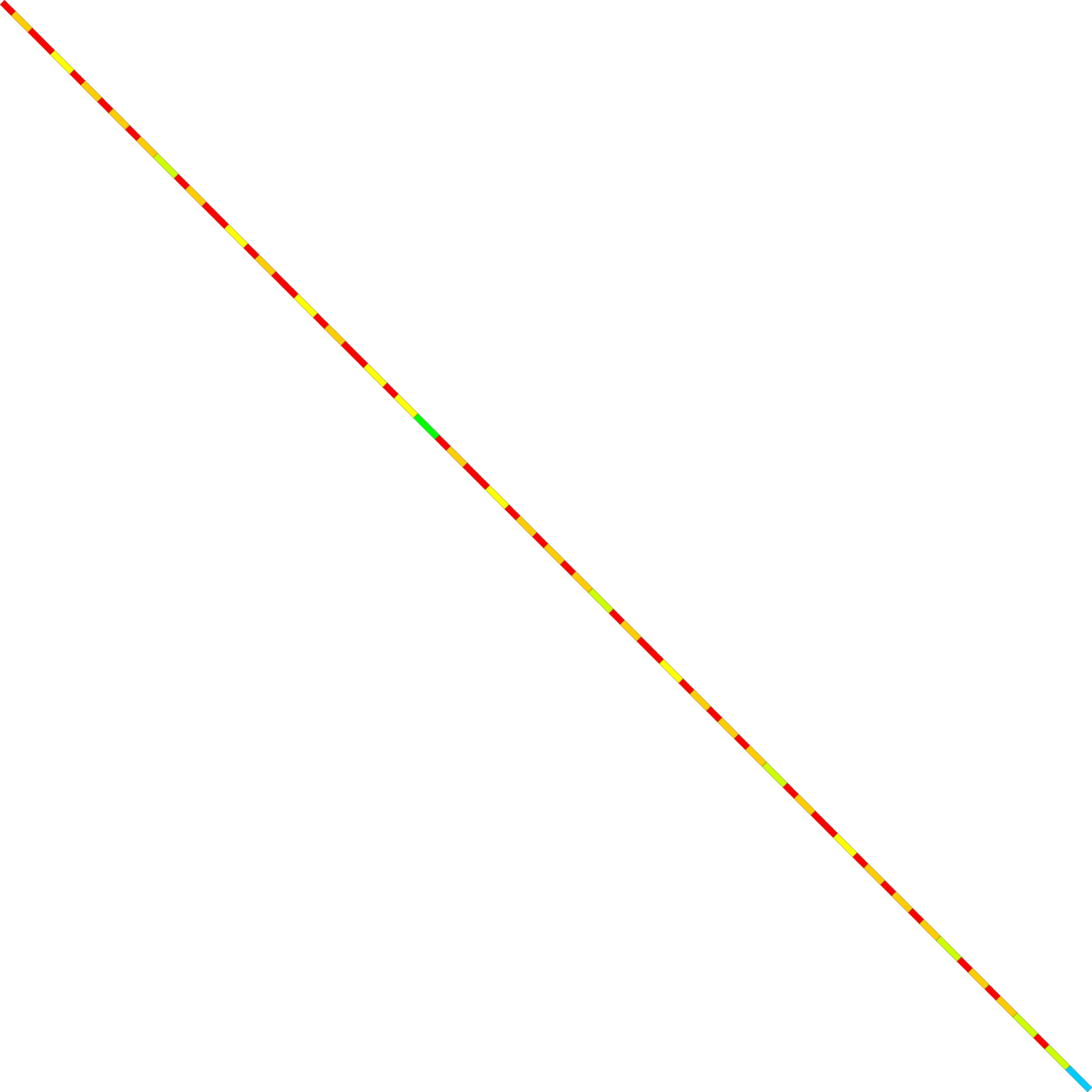## Infinite Number of Proto Tiles

### Info

An one-dimensional substitution rule that uses an infinite number of proto tiles.

The inflation factor is $2.5$.

The substitution rules are given by:

$T_{0}\rightarrow T_{0},T_{1}$

$T_{1}\rightarrow T_{0},T_{0},T_{2}$

$T_{2}\rightarrow T_{0},T_{1},T_{3}$

$T_{k}\rightarrow T_{0},T_{k-1},T_{k+1}$

$T_{\infty}\rightarrow T_{0},T_{\infty},T_{\infty}$

The lengths of the proto tiles are given by:

$length(T_{0})=1$

$length(T_{1})=\frac{3}{2}$

$length(T_{2})=\frac{7}{4}$

$length(T_{k})=\frac{2^{k+1}-1}{2^{k}}$

$length(T_{\infty})=2$

### Substitution Rule### Patchdownload vectorformat Infinite Number of Proto Tiles

### References

[FGM2022]
Frettloeh, D. and Garber, A. and Manibo, N.
Substitution tilings with transcendental inflation factor
2022, 10.48550/ARXIV.2208.01327